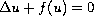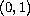Electron. J. Diff. Eqns., Vol. 2006(2006), No. 09, pp. 1-6.

### Nonexistence of solutions to KPP-type equations of dimension greater than or equal to one Janos Englander, Peter L. Simon

Abstract:
In this article, we consider a semilinear elliptic equations of the form, whereis a concave function. We prove for arbitrary dimensions that there is no solution bounded in. The significance of this result in probability theory is also discussed.

Submitted September 19, 2005. Published January 24, 2006.
Math Subject Classifications: 35J60, 35J65, 60J80.
Key Words: KPP-equation; semilinear elliptic equations; positive bounded solutions; branching Brownian-motion.

Show me the PDF file (201K), TEX file, and other files for this article.János Engländer Department of Statistics and Applied Probability University of California Santa Barbara, CA 93106-3110, USA email: englander@pstat.ucsb.edu http://www.pstat.ucsb.edu/faculty/englander Péter L. Simon Department of Applied Analysis, Eötvös Loránd University Pázmány Péter Sétány 1/C, H-1117 Budapest, Hungary email: simonp@cs.elte.hu http://www.cs.elte.hu/~simonp